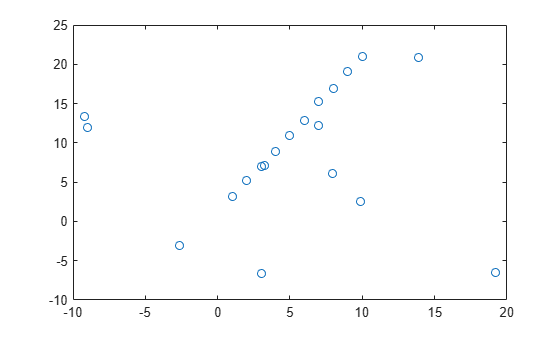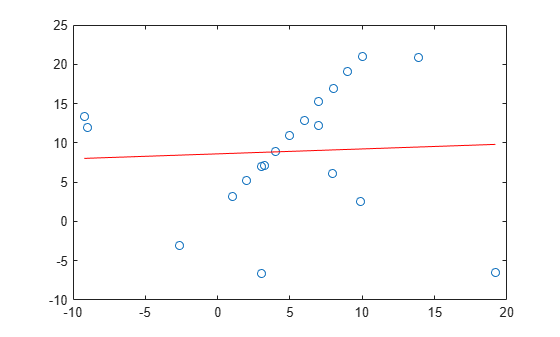# ransac

Fit model to noisy data

## Syntax

``````[model,inlierIdx] = ransac(data,fitFcn,distFcn,sampleSize,maxDistance)``````
``[___] = ransac(___,Name,Value)``

## Description

example

``````[model,inlierIdx] = ransac(data,fitFcn,distFcn,sampleSize,maxDistance)``` fits a model to noisy data using the M-estimator sample consensus (MSAC) algorithm, a version of the random sample consensus (RANSAC) algorithm.Specify your function for fitting a model, `fitFcn`, and your function for calculating distances from the model to your data, `distFcn`. The `ransac` function takes random samples from your `data` using `sampleSize` and uses the fit function to maximize the number of inliers within `maxDistance`.```
````[___] = ransac(___,Name,Value)` additionally specifies one or more `Name,Value` pair arguments.```

## Examples

collapse all

Load and plot a set of noisy 2-D points.

```load pointsForLineFitting.mat plot(points(:,1),points(:,2),'o'); hold on```Fit a line using linear least squares. Due to outliers, the line is not a good fit.

```modelLeastSquares = polyfit(points(:,1),points(:,2),1); x = [min(points(:,1)) max(points(:,1))]; y = modelLeastSquares(1)*x + modelLeastSquares(2); plot(x,y,'r-')```Fit a line to the points using the MSAC algorithm. Define the sample size, the maximum distance for inliers, the fit function, and the distance evaluation function. Call `ransac` to run the MSAC algorithm.

```sampleSize = 2; % number of points to sample per trial maxDistance = 2; % max allowable distance for inliers fitLineFcn = @(points) polyfit(points(:,1),points(:,2),1); % fit function using polyfit evalLineFcn = ... % distance evaluation function @(model, points) sum((points(:, 2) - polyval(model, points(:,1))).^2,2); [modelRANSAC, inlierIdx] = ransac(points,fitLineFcn,evalLineFcn, ... sampleSize,maxDistance);```

Refit a line to the inliers using `polyfit`.

`modelInliers = polyfit(points(inlierIdx,1),points(inlierIdx,2),1);`

Display the final fit line. This line is robust to the outliers that `ransac` identified and ignored.

```inlierPts = points(inlierIdx,:); x = [min(inlierPts(:,1)) max(inlierPts(:,1))]; y = modelInliers(1)*x + modelInliers(2); plot(x, y, 'g-') legend('Noisy points','Least squares fit','Robust fit'); hold off```## Input Arguments

collapse all

Data to be modeled, specified as an m-by-n matrix. Each row corresponds to a data point in the set to be modeled. For example, to model a set of 2-D points, specify the point data as an m-by-2 matrix.

Data Types: `single` | `double`

Function to fit a subset of `data`, specified as a function handle. The function must be of the form:

`model = fitFcn(data)`

If it is possible to fit multiple models to the data, then `fitFcn` returns the model parameters as a cell array.

Function to compute distances from the model to the data, specified as a function handle. The function must be of the form:

`distances = distFcn(model,data)`

If `model` is an n-element array, then distances must be an m-by-n matrix. Otherwise, `distances` must be an m-by-1 vector.

Minimum sample size from `data` that is required by `fitFcn`, specified as a positive scalar integer.

Maximum distance from the fit curve to an inlier point, specified as a positive scalar. Any points further away than this distance are considered outliers. The RANSAC algorithm creates a fit from a small sample of points, but tries to maximize the number of inlier points. Lowering the maximum distance improves the fit by putting a tighter tolerance on inlier points.

### Name-Value Arguments

Specify optional pairs of arguments as `Name1=Value1,...,NameN=ValueN`, where `Name` is the argument name and `Value` is the corresponding value. Name-value arguments must appear after other arguments, but the order of the pairs does not matter.

Before R2021a, use commas to separate each name and value, and enclose `Name` in quotes.

Example: `'MaxNumTrials',2000`

Function to validate model, specified as the comma-separated pair consisting of '`ValidateModelFcn`' and a function handle. The function returns `true` if the model is accepted based on criteria defined in the function. Use this function to reject specific fits. The function must be of the form:

`isValid = validateModelFcn(model,varargin)`

If no function is specified, all models are assumed to be valid.

Maximum number of attempts to find a sample that yields a valid model, specified as the comma-separated pair consisting of '`MaxSamplingAttempts`' and an integer.

Maximum number of random trials, specified as the comma-separated pair consisting of '`MaxNumTrials`' and an integer. A single trial uses a minimum number of random points from `data` to fit a model. Then, the trial checks the number of inliers within the `maxDistance` from the model. After all trials, the model with the highest number of inliers is selected. Increasing the number of trials improves the robustness of the output at the expense of additional computation.

Confidence that the final solution finds the maximum number of inliers for the model fit, specified as the comma-separated pair consisting of '`Confidence`' and a scalar from 0 to 100. Increasing this value improves the robustness of the output at the expense of additional computation.

## Output Arguments

collapse all

Best fit model, returned as the parameters defined in the `fitFcn` input. This model maximizes the number of inliers from all the sample attempts.

Inlier points, returned as a logical vector. The vector is the same length as `data`, and each element indicates if that point is an inlier for the model fit based on `maxDistance`.

 Torr, P. H. S., and A. Zisserman. "MLESAC: A New Robust Estimator with Application to Estimating Image Geometry." Computer Vision and Image Understanding. Vol. 18, Issue 1, April 2000, pp. 138–156.